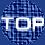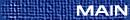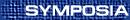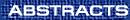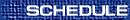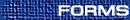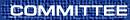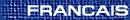Arithmetic Algebraic Geometry / Géométrie algébrique arithmétique
(Kumar Murty and P. Sastry, Organizers)

D. ARAPURA, Purdue University
Hodge cycles on some moduli spaces

I want to give some criteria for the validity of the Hodge conjecture for certain moduli spaces of ``vector bundles'' (actually torsion free sheaves) on curves and surfaces. In the case of curves, the validity of the conjecture for the Jacobian and its powers implies the validity for moduli of higher rank vector bundles. In particular, it holds for generic curves, Fermat curves of prime degree... If time permits, I'd like to say something about the Tate conjectures as well.

B. CONRAD, Department of Mathematics, University of Michigan, Ann Arbor, Michigan  48109-1109, USA
J1(p) has connected fibers

We prove that for every prime p, the minimal regular proper model of X1(p) has geometrically integral closed fiber. Consequently, the component group of J1(p)mod p is trivial. As an application, we can compute the minimal regular proper model of XH(p) = X1(p)/H for any subgroup H of (Z/p)×, and the component group of its Néron model mod p. For example, the map JH(p) ®J0(p) is injective on component groups.

H. DARMON, McGill
To be announced

JORDAN ELLENBERG, Princeton University, Princeton, New Jersey, USA
Pro-p fundamental groups and rational points

A famous circle of conjectures suggests that much of the arithmetic geometry of a variety X over a number field K is encoded in the action of the Galois group of K on the etale fundamental group of X. We discuss some recent results which use the fundamental group of an algebraic curve C to control rational points on C.

E. GOREN, Department of Mathematics and Statistics, McGill University, Montreal, Quebec  H3A 2K6
Hilbert modular varieties of low dimension

I shall discuss Hilbert modular surfaces and threefolds in positive characteristic and their geometry. We use three main tools: local models, displays and Hecke correspondences at p.

ERNST KANI, Queen's University, Kingston, Ontario  K7L 3N6
Hurwitz spaces of genus 2 covers of elliptic curves

Let E/K be an elliptic curve, where K is a field of characteristic ¹ 2. Then the set of (normalized) genus 2 covers f: C® E of E of degree N ³ 3 can be parametrized by smooth curve HE/K,N/K which is an open subset of a twist of the usual modular curve X(N) parametrizing curves with level-N-structure (of fixed determinant).

By studying the degeneration of the universal cover, one can count the total number of genus 2 covers of E/K with fixed discriminant (when K is algebraically closed). This uses the methods of arithmetic geometry: Neron models, minimal models, modular heights and intersection theory.

In addition, one can use the above moduli spaces to study the relation between (certain) modular diagonal quotient surfaces and the Humbert surfaces which are divisors on M2, the moduli space of genus 2 curves.

H. KISILEVSKY, Concordia
To be announced

J. LEWIS, Department of Mathematics, University of Alberta, Edmonton, Alberta  T6G 2G1
K2 of curves and the Mumford-Manin conjecture

We give a formula for a real regulator of the K-groups of a projective algebraic manifold, and apply this to K2 of curves. We explain an application of this to points of finite order on a curve.

YU-RU LIU, Harvard University, USA
Generalizations of the Turán Theorem and the Erdös-Kac Theorem

Let m Î N and w(m) the number of distinct prime divisors of m. The Turán Theorem states

 å m £ x æè w(m) - loglogx öø 2 << x loglog x,
which implies the normal order of w(m) is loglogm. Erdös and Kac give a refinement of this result. For n Î N, they prove

Pn ì
í
î
m: w(m)-loglogm
 Ö loglogm
£ y ü
ý
þ
= ó
õ
y

-¥
e[(-t2)/2] dt,
where Pn is the probability measure that takes place mass 1/n at each m Î N, m £ n. The underlying ideas of these theorems form the foundations of probabilistic number theory. Indeed, the setting of these theorems can be generalized. The main purpose of this talk is to axiomatize the main properties in order to apply the results in a more general context. We will see applications in the cases of number fields, polynomials over a finite field, and smooth projective varieties over a finite field.

R. MURTY, Queen's University
Effective version of Serre's theorem

Let E be an elliptic curve over Q without complex multiplication. For all primes q sufficiently large, Serre proved that the field obtained by adjoing the q-division points to the rationals has Galois group isomorphic to GL2((Fq) where Fq is the finite field of q elements. We will determine an effective lower bound for how large q must be. (This is joint work with Alina Carmen Cojocaru.)

YI OUYANG, University of Toronto, 100 St George St., Toronto, Ontario  M5S 3G3
On the universal norm distribution

We define the universal norm distribution in this talk, which is the generalization of the universal ordinary distribution and the universal Euler system introduced by K. Rubin. Furthermore, a Koszul type resolution (Anderson's resolution) is given to study the universal norm distribution. By this way, we use spectral sequence method to study the group cohomology of the universal norm distribution. Namely, let X be a totally ordered set and let Z be the formal product of X, for any z Î Z, there is an abelian group Gz attached to z. The universal norm distribution Nz is a Gz-module satisfying a certain distribution. When we assign properties to X and Gz, Nz will have abundant arithmetic structures.

IVAN SOPROUNOV, University of Toronto
Residues and tame symbols in toric geometry

We construct an analog of Parshin's theory for toroidal varieties. It turns out to be more explicit than the general theory of Parshin, and is enriched with the combinatorics inherited from toroidal varieties. This approach gives a uniform explanation of recent results of Khovanskii and Gelfond on systems of algebraic equation in (C×)n in terms of symbols and residues on toroidal varieties, and extend these results to the case of an arbitrary algebraically closed field.

Y. ZARHIN, Department of Mathematics, Penn State University, University Park, Pennsylvania  16802, USA
Jacobians with prescribed cyclotomic endomorphism ring

Let p be an odd prime, K a field of characteristic different from p, Ka its algebraic closure, f(x) a polynomial of degree n > 4 with coefficients in K and without multiple roots, C a smooth projective model of the affine curve yp=f(x) and J the jacobian. It is known (Poonen, Schaefer) that the ring End(J) of Ka-endomorphisms of J contains a certain subring isomorphic to the ring of integers Z[mp] in the p-th cyclotomic field Q[mp].

Theorem. Suppose the Galois group of f over K is either the full symmetric group Sn or the alternating group An. Then:

1) If K has characteristic zero then End(J)=Z[mp].
2) If n > 9 and p divides n then Z[mp] is a maximal commutative subring in End(J).